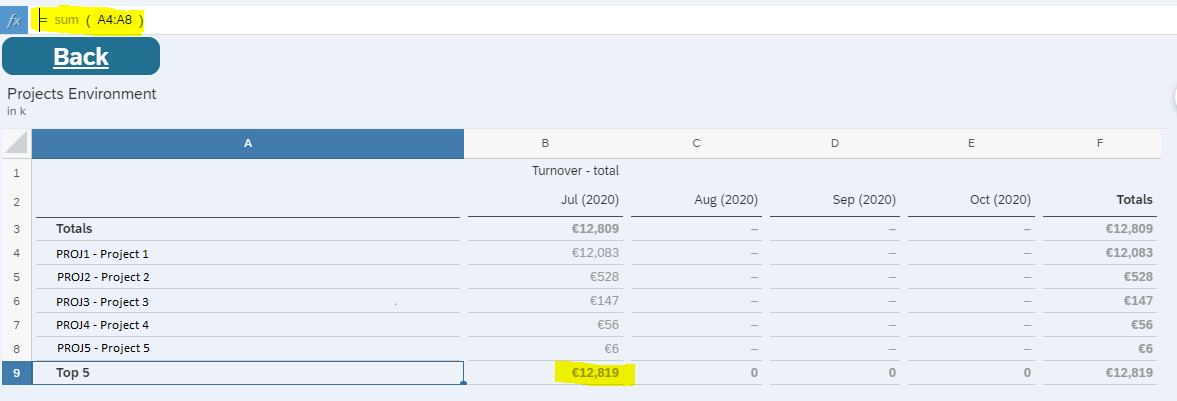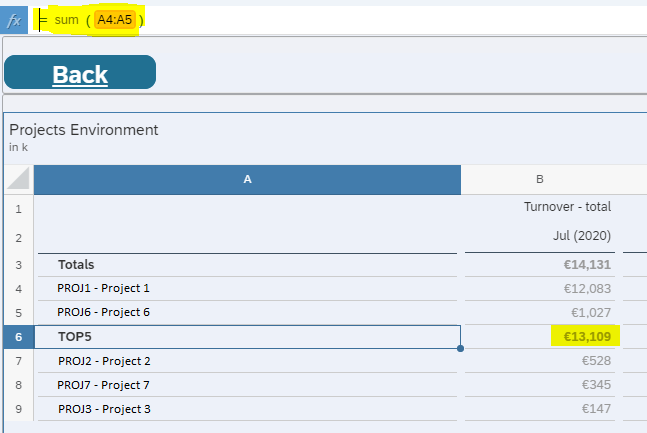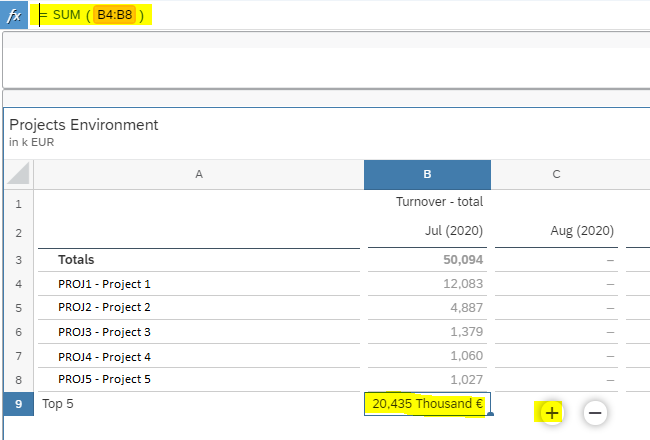# SAP Analytics Cloud Tips - Solve corrupt formulas when using front-end calculations

### Challenge

When using front-end calculations in tables in SAP Analytics Cloud (SAC), it might happen that these formulas get corrupted or even completely disappear after the content of the table changes. For example, if you want to take the sum of 5 lines in a table, you can add a new line and add the formula directly on the label (A9) as shown below.
This formula will sum the data of row 4 to row 8 (sum(A4:A8)). Adding the formula on the label, will calculate for the entire row and will work, only if the rows inside the table remain the same.After the context and the content of the table changes, the formula is not correct anymore. It shifted position and the formula is not correct. In some cases, the formula will change to REF_ERR, indicating a reference error. This is because calculations added to the rows makes fixed references to the members used in the table. Some members are not anymore in the table or have shifted in position.### Solution

To overcome this issue the trick is to use cell-based calculations instead. The main difference is that cell-based calculations are not valid for the entire row or column but only for the cell in which we put the formula. As a result, the formula will not be assigned to the label of the row/column but on the cell itself by doing the following actions:

1. Click on the + on the bottom of the table to add a new line to the table
2. Select cell A9 and type a label in the rows as in this example (Top 5)
3. Do not put a formula for the entire row but put the formula directly in the cell. (Cell B9)
4. Use SUM() in the formula as SUM does not make fixed references to the members in the rows. Direct references to the cells (B2 + B3 + …) will make fixed references to the members. So, if the content of the table changes, this will result in REF_ERR.
5. Repeat step 3 and 4 for each cell where the formula should be present

When the content of the table will change, the “Top 5” row will remain on the correct position and the formula will remain correct.```SAP Analytics Cloud version used in this article:
System Name 6EA70
Version 2020.22.1
Build Date Wed Nov 18 2020 20:05:08+0100```